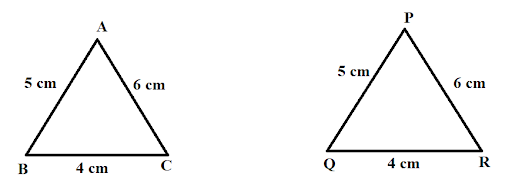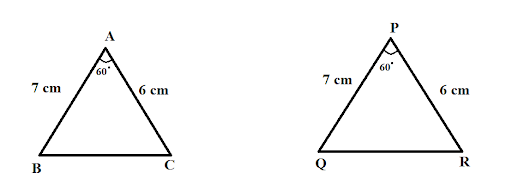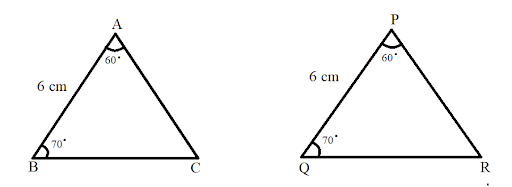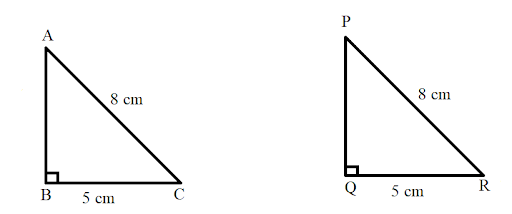Courses
Courses for Kids
Free study material
Offline Centres
More

# Congruence of Triangles Class 7 Notes CBSE Maths Chapter 7 (Free PDF Download)Last updated date: 07th Dec 2023
Total views: 593.4k
Views today: 15.93k

## Revision Notes for CBSE Class 7 Maths Chapter 7 - Free PDF Download

Discover a valuable resource for Class 7 Mathematics with free PDF downloads of revision notes and key-notes for Chapter 7 - Congruence of Triangles. Our expert mathematics teachers have prepared these materials using the latest CBSE (NCERT) books, making it easier for you to understand and grasp the concepts effectively. Should you ever have questions or doubts, don't hesitate to register for mathematics tuitions on Vedantu.com. Our platform is dedicated to helping you clear any confusion, ensuring you can approach your studies with confidence, whether you're preparing for an upcoming exam or seeking a better understanding of the subject.

Also, check CBSE Class 7 Maths revision notes for All chapters:

## Access Class 7 Mathematics Chapter 7 – Congruence of Triangles Notes

### Congruence

• Two object is said to be congruent if and only if their shape and size are same.

• The relation between two congruent object is called congruence.

• Congruence is denoted by symbol $\cong$

### Congruence of Plane Figures

• Two plane figures are said to be congruent when two plane figures superpose each other i.e., when one figure is placed on another figure they coincide.

• Suppose a plane ${{P}_{1}}$ is congruent to plane ${{P}_{2}}$ then it is written or denoted as ${{P}_{1}}\cong {{P}_{2}}$

### Congruence Among Line Segments

• Two lines are said to be congruent if they have equal length.

• Conversely, if two lines are congruent, they are of equal length.

• Suppose a line $\overline{AB}$ is congruent to plane $\overline{CD}$ then it is written or denoted as $\overline{AB}\cong \overline{CD}$

### Congruence of Angle

• Two angles are said to be congruent if they have equal measure.

• Conversely, if two angles are congruent, they are of equal measure.

• Suppose an angle $\angle ABC$ is congruent to angle $\angle CDE$ then it is written or denoted as $\angle ABC\cong \angle CDE$

### Congruence of Triangle

• Two triangles are said to be congruent if they have corresponding vertices, corresponding sides and corresponding angles equal.

• Let us suppose two triangles $\Delta ABC$ and $\Delta PQR$ has correspondence as

$A\leftrightarrow P$

$B\leftrightarrow Q$

$C\leftrightarrow R$

It can be written as $ABC\leftrightarrow PQR$ which implies that $\Delta ABC\cong \Delta PQR$

• In the above case if we denote it as $\Delta ABC\cong \Delta PRQ$ then it is not possible since vertex $B$ is corresponding to vertex $Q$ not $R$

• Therefore, congruence should be denoted in proper way since the order of the letters in the names of congruent triangles displays the corresponding relationships.

### Criteria For Congruence of Triangle

• There are four criteria for congruence of triangle and these are

1. SSS Congruence Criteria

• If under a given correspondence, the three sides of one triangle are equal to the three corresponding sides of another triangle, then the triangles are congruent.

• For example:In triangles $\Delta ABC$ and $\Delta PQR$

$AB=PQ$

$BC=QR$

$AC=PR$

Then by SSS congruency $\Delta ABC\cong \Delta PQR$

1. SAS Congruence Criteria

• If under a correspondence, two sides and the angle included between them of a triangle are equal to two corresponding sides and the angle included between them of another triangle, then the triangles are congruent.

• For exampleIn triangles $\Delta ABC$ and $\Delta PQR$

$AB=PQ$

$\angle BAC=\angle QPR$

$AC=PR$

Then by SAS congruency $\Delta ABC\cong \Delta PQR$

1. ASA Congruence Criteria

• If under a correspondence, two angles and the included side of a triangle are equal to two corresponding angles and the included side of another triangle, then the triangles are congruent.

• For exampleIn triangles $\Delta ABC$ and $\Delta PQR$

$AB=PQ$

$\angle BAC=\angle QPR$

$\angle ABC=\angle PQR$

Then by ASA congruency $\Delta ABC\cong \Delta PQR$

1. RHS Congruence Criteria

• If under a correspondence, the hypotenuse and one side of a right-angled triangle are respectively equal to the hypotenuse and one side of another right-angled triangle, then the triangles are congruent.

• This criterion is applicable on right angle triangles only.

• For exampleIn triangles $\Delta ABC$ and $\Delta PQR$

$AC=PR$

$BC=QR$

Then by RHS congruency $\Delta ABC\cong \Delta PQR$

## What are the Benefits of Referring to Vedantu’s Revision Notes for Class 7 Chapter 7 - Congruence of Triangles

• Provides quick, clear summaries of key concepts.

• Simplifies complex topics for better understanding.

• Efficient tool for last-minute exam prep.

• Enhances retention of crucial information.

• Supports effective exam preparation with key points and tips.

• Saves time by consolidating information.

• Prioritizes important topics and questions.

• Offers practical examples for real-world connections.

• Boosts student confidence for exams.

## Conclusion

For an enhanced comprehension of this subject, NCERT - Class 7 Chapter 7 - Congruence of Triangles thoughtfully prepared by experienced educators at Vedantu is your invaluable companion. These resources break down the complexities of Class 7 Chapter 7 - Congruence of Triangles into easily digestible sections, helping you grasp new concepts, master formulas, and navigate through questions effortlessly. By immersing yourself in these materials, you not only prepare for your studies more efficiently but also develop a profound understanding of the subject matter.

## FAQs on Congruence of Triangles Class 7 Notes CBSE Maths Chapter 7 (Free PDF Download)

1. Are NCERT Solutions for Class 7 Maths Chapter 7 important from an exam point of view?

Yes, NCERT solutions of Class 7 Maths Chapter 7 is important from an exam point of view as these questions will make your exam preparation easier and you will get accurate solutions to all the questions. You can prepare from those questions while you are revising for your exam and cross-check all the solutions so that you do not write wrong answers in your exams. The solutions are accurate and you can rely on them.

2. Give me a summary on NCERT Solutions for Class 7 Maths Chapter 7?

This chapter is a congruence of triangles. It has two exercises that are related to different topics. The main topics include:

• Plain figure’s congruence

• Congruence inline segments

• Congruence in triangle and angles

• Right-angled triangle’s congruence

For more information, visit Vedantu’s official website. There you will find the solved answers which will be helpful in your exams. These solutions will cover all the important topics which are curated together from the exam's point of view. You can download these questions and save them on your PC for future reference.

3. What will students learn after solving questions from this chapter?

Students will appreciate the usefulness of superposition after going through the fundamental ideas of congruence outlined in this chapter. Superposition is a useful means of assessing whether any two-plane figures are congruent. Students can try applying the congruence requirements for line segments, angles, and triangles to different planar figures after learning about them. When establishing that two triangles are congruent, there are a few theorems that must be taken into account. In NCERT solutions Class 7 maths chapter 7, they are logically proven and discussed.

4. What are the criteria for the congruence of triangles according to Class 7?

They are called congruent when the three angles of one triangle are equal to the three angles of the other triangle. This is the SSS rule. When two angles and one side of the triangle are similar to the other triangle then it is called the ASA rule. When two angles and other angles of one triangle are equal to the other sides of another triangle then it is called the SAS rule.

5. Is it necessary for me to go over all of the questions in NCERT Solutions Class 7 Maths Congruence of Triangles?

The NCERT Solutions Class 7 Maths Congruence of Triangles properly explains the triangles, which are the beginning of the geometry subject. As a result, students will benefit from regular practice of the exercise problems to have a clear grasp of all of the principles and properties of triangles, which will ensure a solid basis for knowledge of future geometry topics. The CBSE board always recommends these answers to students for test preparation because they are simple to obtain and understand.